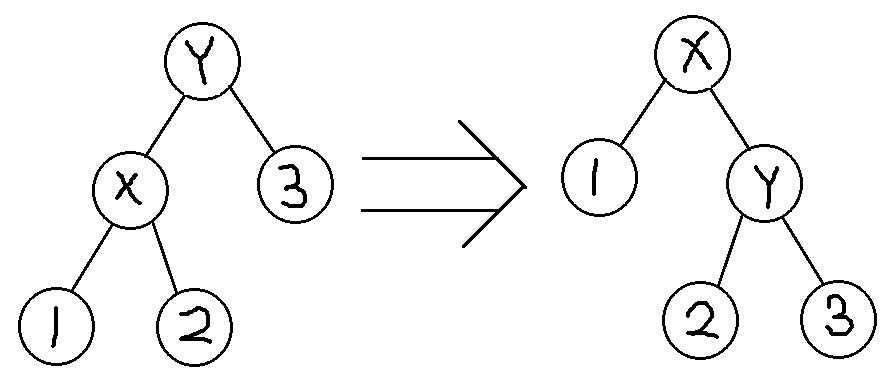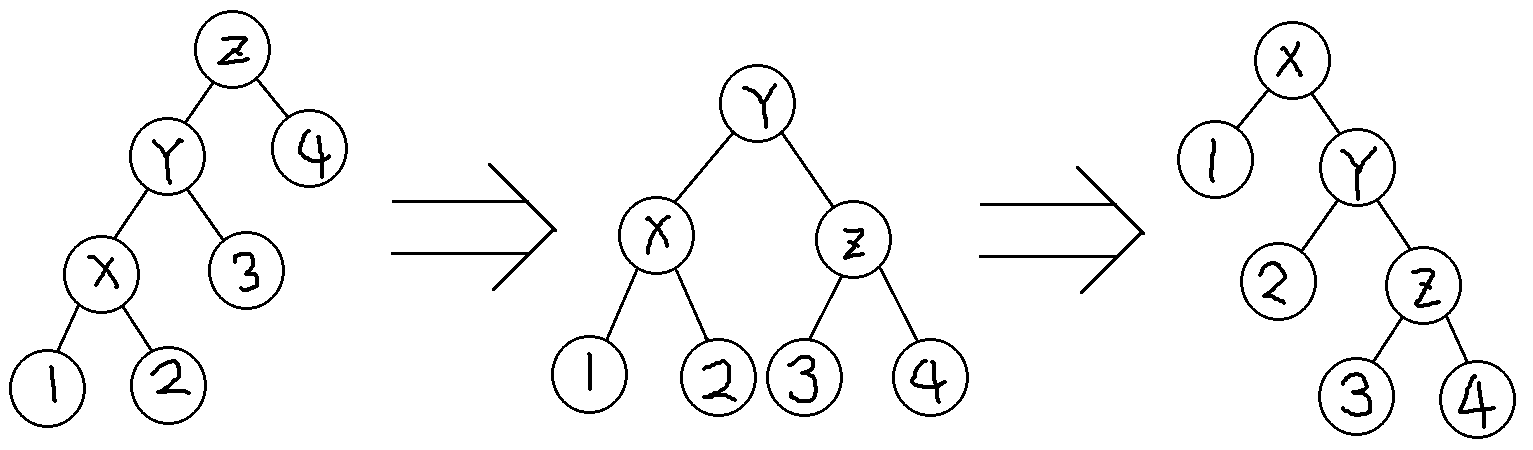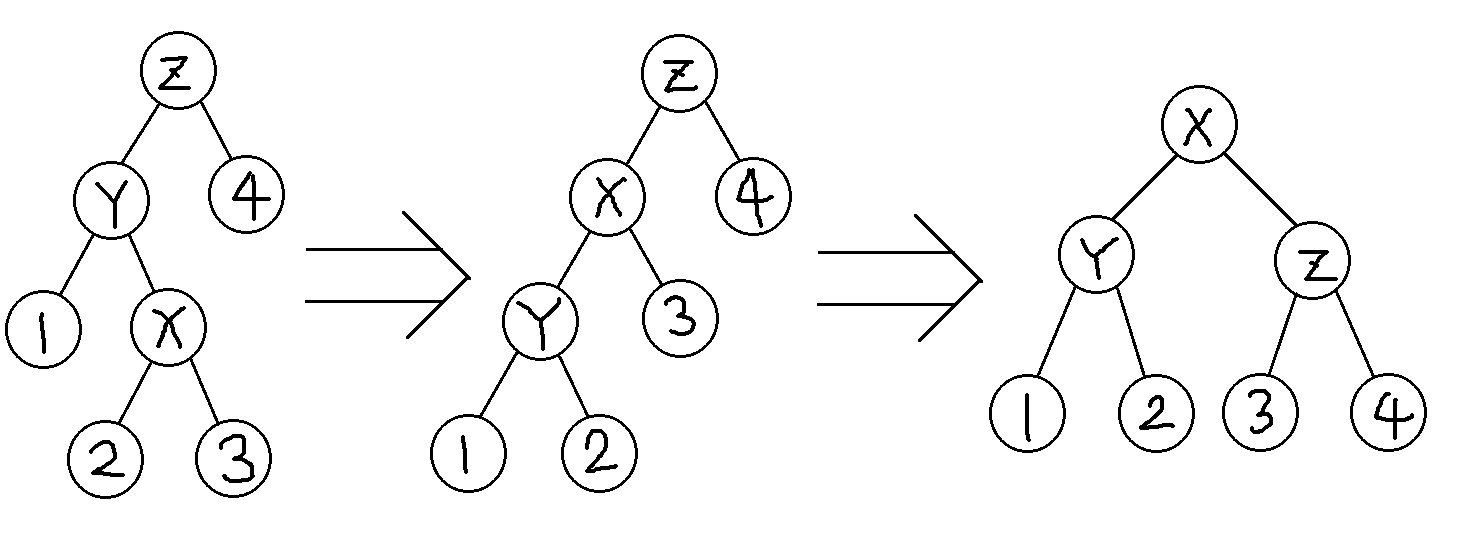1. 引入

2. 简单例子

3. 证明 splay 复杂度

4. 证明 LCT 复杂度

# 引入

为什么 KMP 不能可持久化，而要用 KMP 自动机来代替？

为什么 splay 不能可持久化，仅仅只是因为难以维护 father 指针吗？

答案是——它们都是基于均摊分析的。

均摊时间复杂度是什么？

这里有一个容易混淆的概念：期望时间复杂度。

期望时间复杂度是指在随机情况下，算法的每种时间复杂度乘上它发生的概率之和。

而均摊时间复杂度不一样。它是指总复杂度在一个范围内，但是单次操作的复杂度并不是总复杂度除以操作数，甚至有可能接近总复杂度。

本文主要部分参照陈胤伯的《均摊分析简介》改写。

# 简单例子

## 问题

一个初始值为 0 的 k 位二进制计数器，每次加一。每次加一的运算次数为修改的位数。请问时间复杂度是什么？

## 证明与答案

作为一个简单例子，自然有很多简单证法。

例如，这个 k 位二进制数第 i 位，需要每加 $2^i$ 次才会变一次。所以所有位的变化次数之和为

$$\sum_i \frac{n}{2^i} = O(n)$$

所以单词操作均摊 $O(1)$ 。

接下来，引入一种更通用的方法——势能分析。

定义势能函数 $\phi$ 表示定义域当前状态的一个函数。

定义 $\phi(i)$ 为第 i 次操作后的状态的势能函数值。

定义第 i 次操作的实际消耗时间为 $t_i$ 。

定义第 i 次操作的均摊时间话费为 $a_i$ ，$a_i = t_i + \phi(i) - \phi(i-1)$ 。

为了方便书写，如不加说明，则用 $\Delta phi$ 表示 $\phi(i)-\phi(i-1)$ 。

于是我们可以用上述定义得到总时间复杂度为

$$\phi(0)-\phi(n)+\sum_{i=1}^{n} a_i$$

接下来回到原先的例子：

定义 $\phi$ 表示当前 k 位二进制数中值为 1 的位的个数，显然第 i 次操作有一个 0 变成 1，设第 i 次操作有 x 个 1 变成 0 。那么

$$a_i=t_i+\Delta\phi = (1+x) + (1-x) = 2$$

所以总时间复杂度为

$$\phi(n) - \phi(0) + \sum_{i=1}^n a_i \leq k + 2n = O(k+n)$$

朴素地讲，我们可以把势能函数看做自己的存款，在耗时的时候消耗以换取时间，在不耗时的时候存储。只要总的变化量在一定范围内，那么总的复杂度就可以被接受。

# 证明 Splay 的复杂度

相信经过一定的思考，你会发现势能分析的一个关键在于确定合适的势能函数 $\phi$ 。接下来，在证明 splay 复杂度的过程中，你将会体验到势能分析的另一个关键——合理放缩。

## 定义

设 splay 树的大小和操作次数都是 $O(n)$ 。

定义 $size[x]$ 表示节点 x 的子树大小。

定义函数 $f(x) = \log_2 (size[x])$，接下来简写为 $f(x) = \log (size[x])$ 。注意这个底数 2 是不能忽视的。

定义 $f'(x)$ 表示本次操作之后的 $f(x)$ 。

定义 $\phi = \sum f(x)$ 。

splay 的操作可以分为单旋和双旋，这里定义单次单旋或者双旋的均摊时间花费为 $S(x)$ 。

## 证明

我们来给旋转操作分成 3 类：

1. 单旋；

2. 双旋 - 先旋 father ，再旋 x；

3. 双旋 - 旋转 x 两次 。

我们来依次证明三种旋转操作的复杂度。下面默认对节点 x 做旋转操作。

### 单旋$$\begin{eqnarray*}S(x) &=& 1 + f'(x) + f'(y) - f(x) - f(y) \\ &=& 1 + f'(y) - f(x) \\ &\leq & 1 + (f'(x) - f(x))\end{eqnarray*}$$

### 双旋 - 先旋 father ，再旋 x$$\begin{eqnarray*}S(x) &=& 2 + f'(x) + f'(y) + f'(z) - f(x) - f(y) - f(z) \\ &=& 2 + f'(y) + f'(z) - f(y) - f(x) \\ &\leq & 2 + f'(x) + f'(z) - 2f(x) \end{eqnarray*}$$

$$\begin{eqnarray*}f(x) + f'(z) - 2f'(x) &=& \log_2\left(\frac{size[x] \cdot size'[z]}{size'[x] ^2 }\right)\end{eqnarray*}$$

$$size[x] + size'[z] = size + size + size + size + 2 \leq size'[x]$$

$$size[x] \cdot size'[z] \leq \frac 1 4 size'[x] ^ 2$$

$$f(x) + f'(z) - 2f'(x) \leq \log_2 (\frac 1 4) = -2$$

$$-2 - (f(x) + f'(z) - 2f'(x))\geq 0{\tag 1}$$

$$S(x) \leq 2 + f'(x) + f'(z) - 2f(x) + (-2 - (f(x) + f'(z) - 2f'(x))) = 3(f'(x) - f(x))$$

### 双旋 - 旋转 x 两次$$\begin{eqnarray*}S(x) &=& 2 + f'(x) + f'(y) + f'(z) - f(x) - f(y) - f(z) \\ &=& 2 + f'(y) + f'(z) - f(y) - f(x) \\ &\leq & 2 + f'(y) + f'(z) - 2f(x) \end{eqnarray*}$$

$$-2-(f'(y) + f'(z)-2f'(x))\geq 0$$

$$S(x) \leq 2 + f'(y) + f'(z) - 2f(x) + (-2-(f'(y) + f'(z) - 2f'(x))) = 2(f'(x) - f(x))$$

$$1 + (f'(x) -f(x))\\ 3(f'(x) - f(x)) \\ 2(f'(x) - f(x))$$

$$T(n) = \sum_{i=1}^n a_i + \phi(0) - \phi(n) \leq O(n\log n)$$

P.S. 对splay做切割与合并操作时，只要先把连接点旋到根就容易发现势能函数发生变化的节点数是 $O(1)$ 的。

# 证明 LCT 的复杂度

## 定义

LCT 的证明和 Splay 类似。这里我们将 $size[x]$ 的定义稍加修改，改成辅助树上 子树 x 的大小。

## 证明

LCT中splay部分的证明和 Splay 的复杂度证明一样。

这里只考虑虚实链切换时的复杂度。

如果 size[x]*2 >= size[fa[x]] ，那么我们称 x 为 fa[x] 的重儿子，fa[x] 的其他儿子为轻儿子。

那么，显然一个节点在 access 的过程中只会遇见 $O(\log n)$ 个轻儿子。

定义势能函数 $\delta$ 表示实儿子和重儿子不同的节点个数。

考虑一次 access 。

考虑一次 splay 后，当前splay根节点 x 的情况：

1. 如果它是轻儿子，那么消耗 1 时间，势能函数增量小于等于 1 。

2. 如果它是重儿子，那么消耗 1 时间，势能函数减1 。

所以一次access 在轻重链切换上消耗的均摊时间就等于 1. 操作的均摊时间花费，所以一次access的均摊时间复杂度为 $O(\log n)$ 。

所以 $LCT$ 的时间复杂度是

$$O(n\log n)$$

### 换根

考虑将一个连通块的根从 $x$ 变更为 $y$ 时，哪些边的轻重发生了改变。我们发现，可能改变轻重的边只有 $x$ 到 $y$ 路径上的边，而改变前和改变后这条路径上均只有 $O(\log n)$ 个轻边，所以变化量为 $O(\log n)$。

考虑 Cut 操作：假设在以 $y$ 为根的连通块上切除子树 $x$，我们发现影响到的也只有 $x$ 到 $y$ 路径上的边，同理变化量还是 $O(\log n)$。
考虑 Link 操作：假设将根为 $x$ 的连通块和根为 $y$ 的连通块通过某两个点相连，我们发现，如果我们取 $x$ 或者 $y$ 为新连通块的根，那么影响到的仍然是连边后 $x$ 到 $y$ 路径上的边，同理变化量还是 $O(\log n)$。